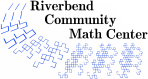# Nim Games

### Description

Try these counting games with a friend. Can you figure out a strategy so that you can win no matter what your opponent does?

### Levels

• Adults will find this activity interesting.

0 minutes

5 to 60 minutes

### Topics

• subtraction
• multiplication
• division
• modular arithmetic

### Goals

• Students will understand the strategic role of the ``winning numbers'' in counting games.
• Students will identify patterns in the winning numbers. Younger students will identify these patterns in terms of counting or subtraction. Older students will identify these patterns in terms of multiples and remainders.
• Students will adapt strategies learned in one game to slightly different games.
• Students will generalize strategies learned in similar games to a general strategy for games of this type.
• Students will increase their comfort level with expressing mathematical ideas by using relevant math terms to record their strategies.
• Some game variations can lead to deeper mathematical insights.

• counting

• multiplying
• division

### Related Concepts

• modular arithmetic

### Credits

• Some variations come from the files of Tatiana Shubin and Paul Zeitz.
• ``Cartesian Chase'' comes from Thinking Mathematically by Mason, Burton, and Stacey, page 162.
• Steve Dingledine contributed the ``Adding Up To 100'' variation.
• Phil Yasskin shared ``The Rolling Die'' game, which he saw at a Renaissance Festival.

### Author

Amanda Katharine Serenevy

### National Common Core Standards

 1.OA.5 Relate counting to addition and subtraction (counting on / counting back / counting up strategies). 1.OA.6 Add and subtract within 20, demonstrating fluency for addition and subtraction within 10. (Use strategies such as counting on, counting back, counting up, making ten, decomposing, using fact families). 2.Focus.2 Build fluency with addition and subtraction. 2.OA.2 Fluently add and subtract within 20 using mental strategies. By end of Grade 2, know from memory all sums of two one-digit numbers. 3.Focus.1 Develop understanding of multiplication and division and strategies for multiplication and division within 100. 3.OA.7 Fluently multiply and divide within 100, using strategies such as the relationship between multiplication and division. By the end of third grade, memorize all products of one-digit numbers. 3.OA.9 Identify arithmetic patterns including patterns in the addition or multiplication table, and explain them using properties of operations. 4.OA.3 Solve multistep word problems posed with whole numbers and having whole-number answers using the four operations, including problems in which remainders must be interpreted. 4.OA.5 Generate a number or shape pattern that follows a given rule. Identify apparent features of the pattern that were not explicit in the rule itself. (For example, notice that when generating numbers by successively adding three that evens alternate with odds, and reason about why.) K.CC.1 Count to 100 by ones and tens. K.CC.2 Count forward from a given number instead of beginning at 1. K.CC.4 Connect counting to cardinality. (Count objects and understand that numbers refer to quantities.) K.Focus.1 Represent, relate, and operate on whole numbers.
Riverbend Community Math Center
hello@riverbendmath.org
http://riverbendmath.org
(574) 339-9111This work placed into the public domain by the Riverbend Community Math Center.